Скачать презентацию 12 3 Efficient Diversification with Many Assets

d2754fd1e1e41b8cf2f640c7dbee2c03.ppt

• Количество слайдов: 39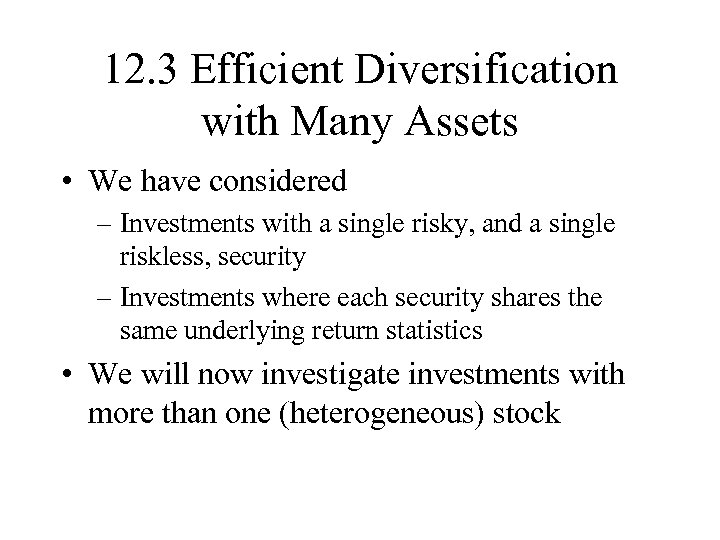12. 3 Efficient Diversification with Many Assets • We have considered – Investments with a single risky, and a single riskless, security – Investments where each security shares the same underlying return statistics • We will now investigate investments with more than one (heterogeneous) stock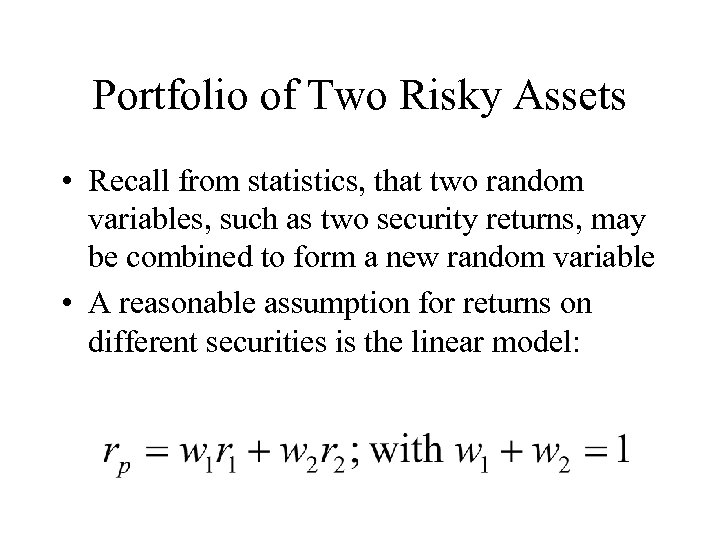Portfolio of Two Risky Assets • Recall from statistics, that two random variables, such as two security returns, may be combined to form a new random variable • A reasonable assumption for returns on different securities is the linear model: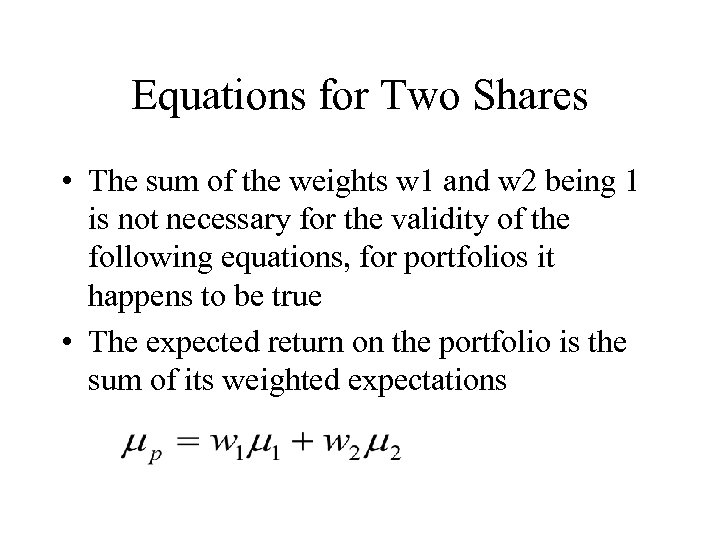Equations for Two Shares • The sum of the weights w 1 and w 2 being 1 is not necessary for the validity of the following equations, for portfolios it happens to be true • The expected return on the portfolio is the sum of its weighted expectations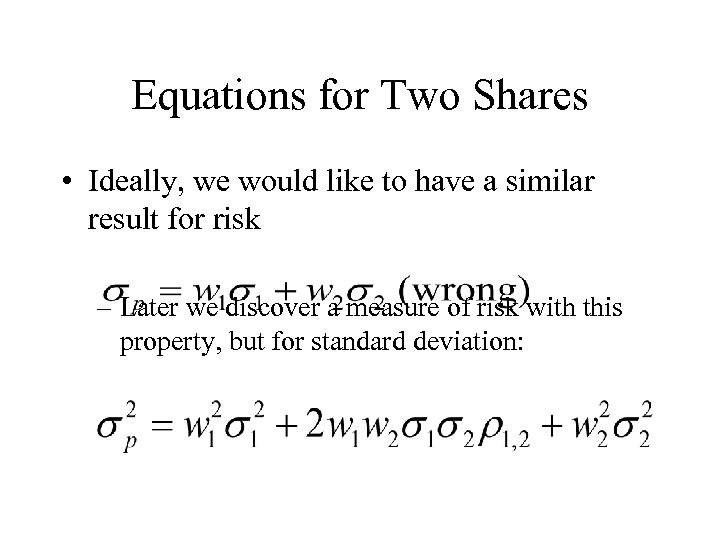Equations for Two Shares • Ideally, we would like to have a similar result for risk – Later we discover a measure of risk with this property, but for standard deviation: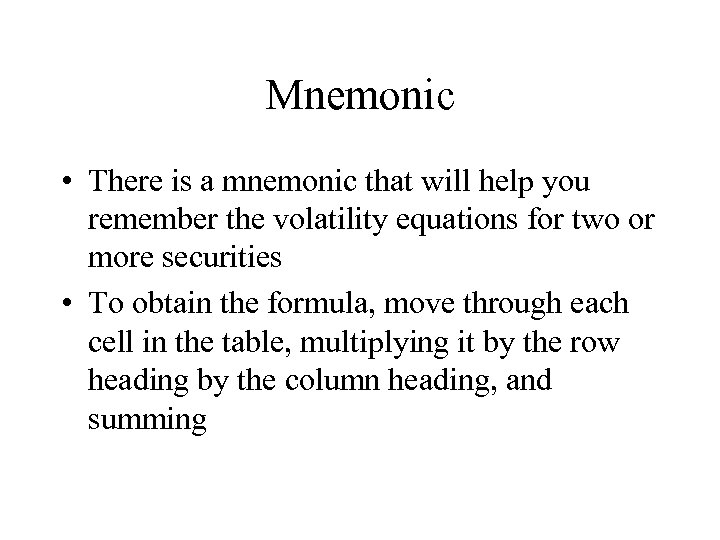Mnemonic • There is a mnemonic that will help you remember the volatility equations for two or more securities • To obtain the formula, move through each cell in the table, multiplying it by the row heading by the column heading, and summing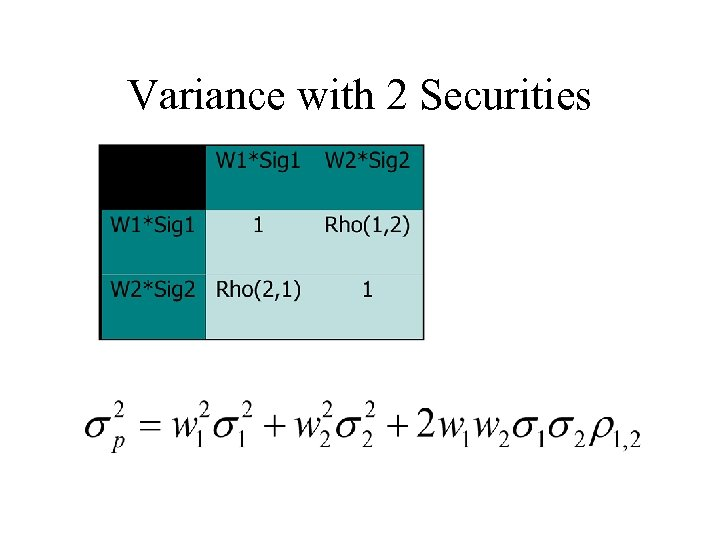Variance with 2 Securities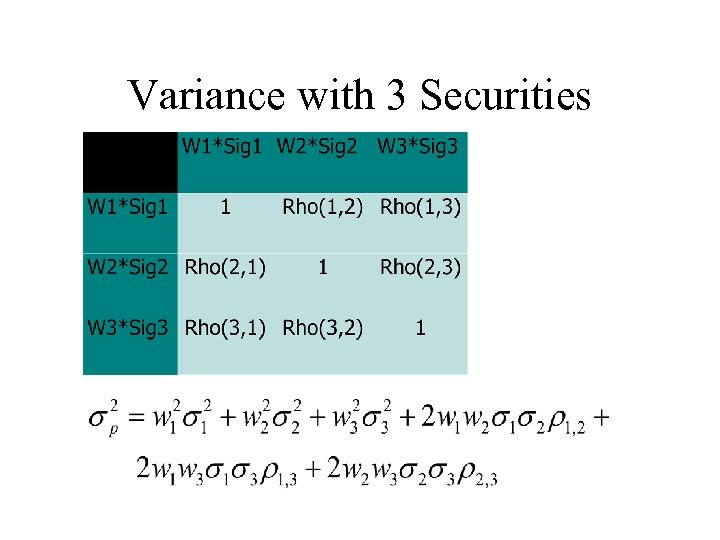Variance with 3 Securities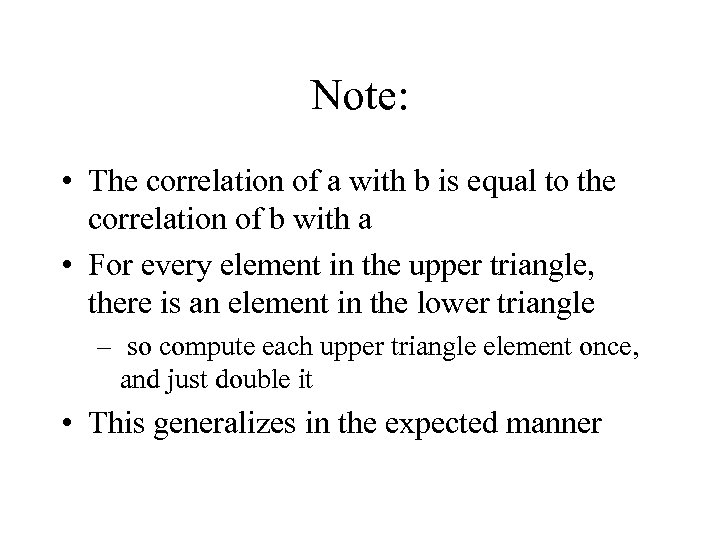Note: • The correlation of a with b is equal to the correlation of b with a • For every element in the upper triangle, there is an element in the lower triangle – so compute each upper triangle element once, and just double it • This generalizes in the expected manner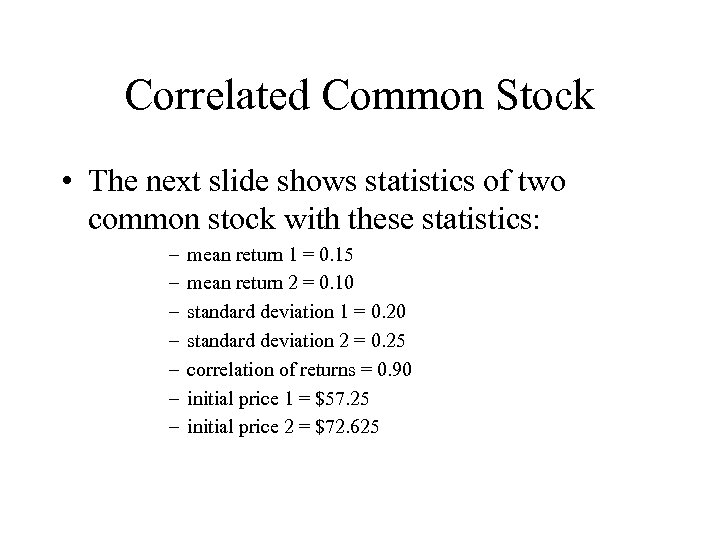Correlated Common Stock • The next slide shows statistics of two common stock with these statistics: – – – – mean return 1 = 0. 15 mean return 2 = 0. 10 standard deviation 1 = 0. 20 standard deviation 2 = 0. 25 correlation of returns = 0. 90 initial price 1 = \$57. 25 initial price 2 = \$72. 625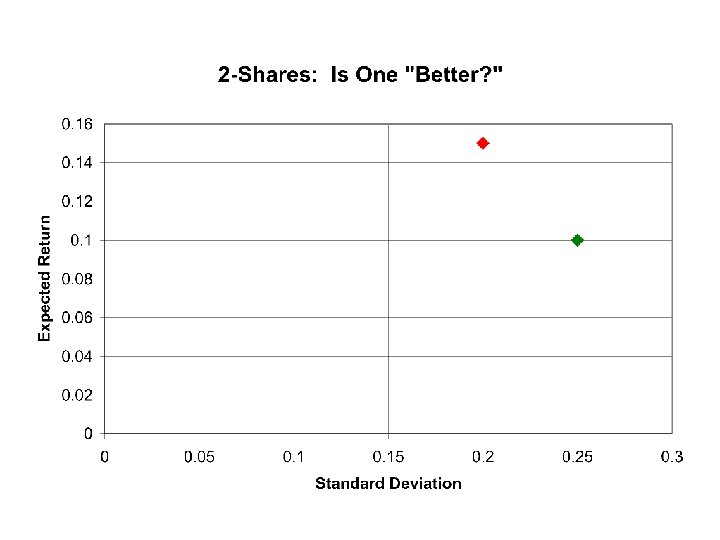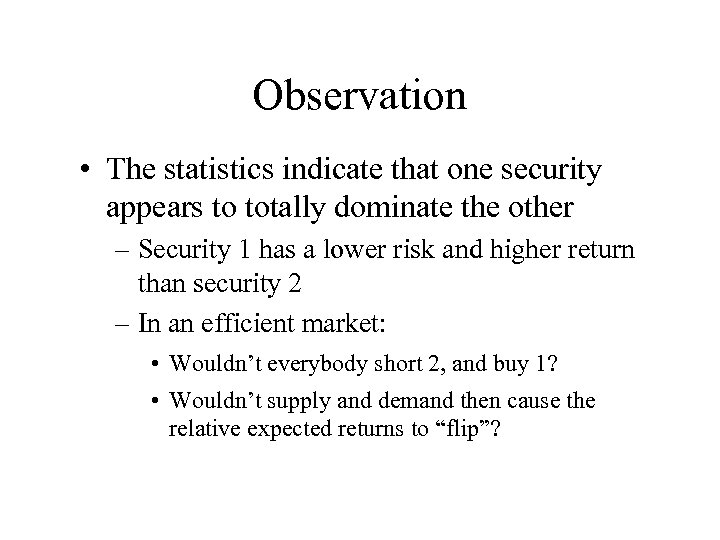Observation • The statistics indicate that one security appears to totally dominate the other – Security 1 has a lower risk and higher return than security 2 – In an efficient market: • Wouldn’t everybody short 2, and buy 1? • Wouldn’t supply and demand then cause the relative expected returns to “flip”?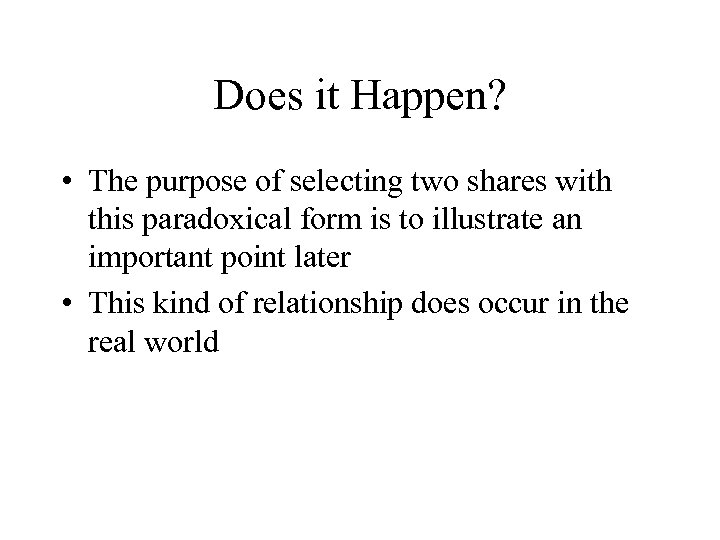Does it Happen? • The purpose of selecting two shares with this paradoxical form is to illustrate an important point later • This kind of relationship does occur in the real world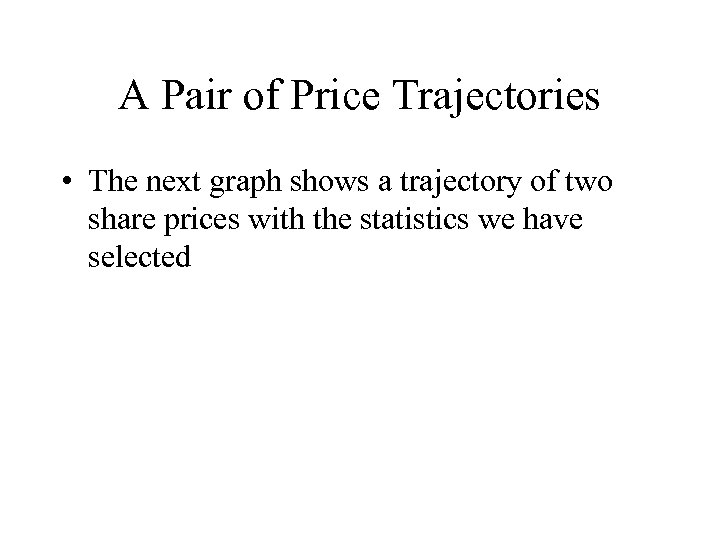A Pair of Price Trajectories • The next graph shows a trajectory of two share prices with the statistics we have selected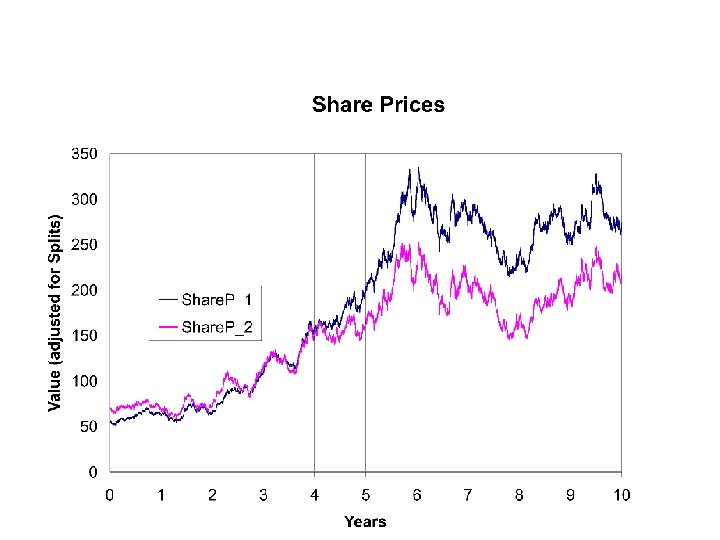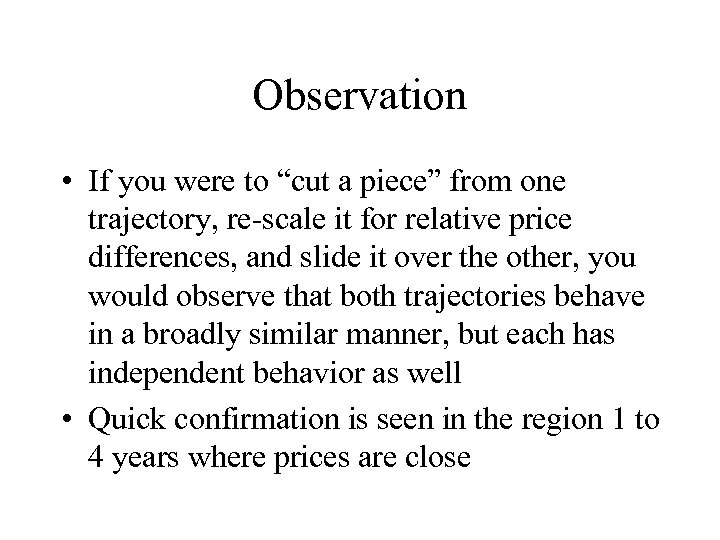Observation • If you were to “cut a piece” from one trajectory, re-scale it for relative price differences, and slide it over the other, you would observe that both trajectories behave in a broadly similar manner, but each has independent behavior as well • Quick confirmation is seen in the region 1 to 4 years where prices are close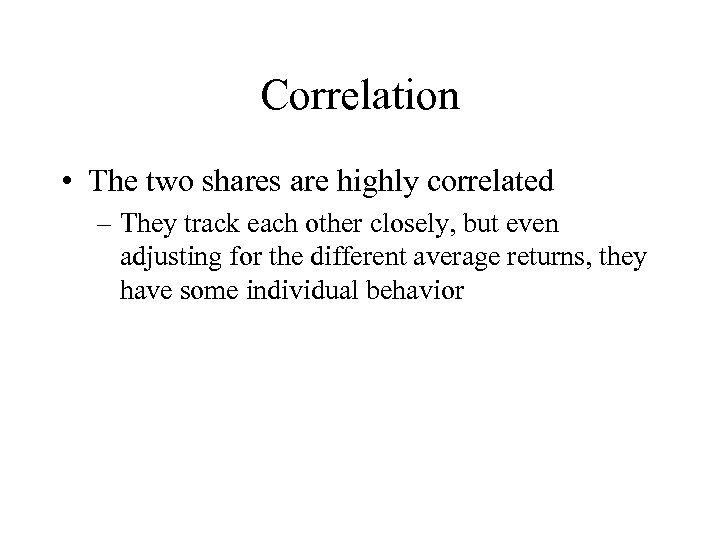Correlation • The two shares are highly correlated – They track each other closely, but even adjusting for the different average returns, they have some individual behavior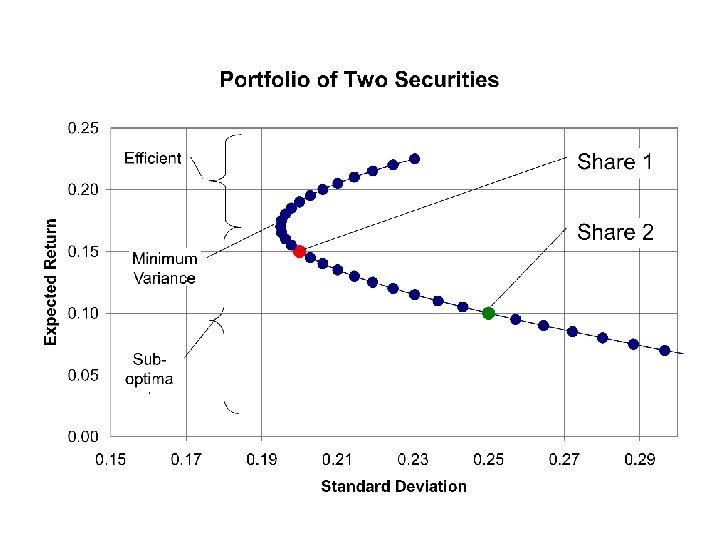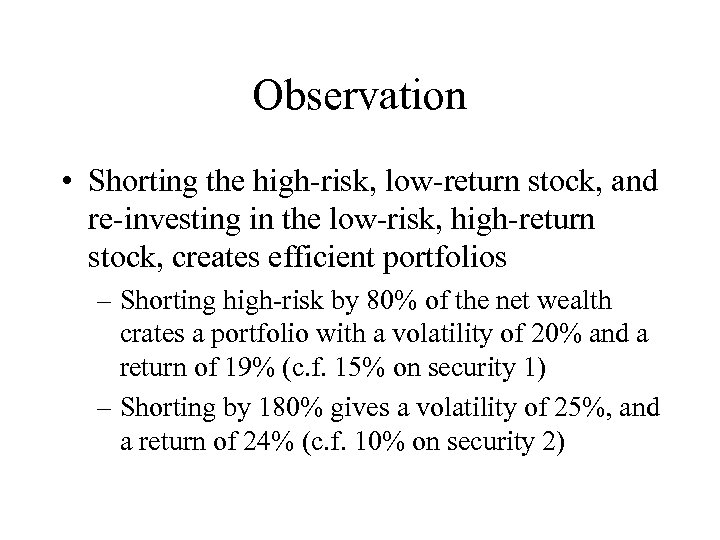Observation • Shorting the high-risk, low-return stock, and re-investing in the low-risk, high-return stock, creates efficient portfolios – Shorting high-risk by 80% of the net wealth crates a portfolio with a volatility of 20% and a return of 19% (c. f. 15% on security 1) – Shorting by 180% gives a volatility of 25%, and a return of 24% (c. f. 10% on security 2)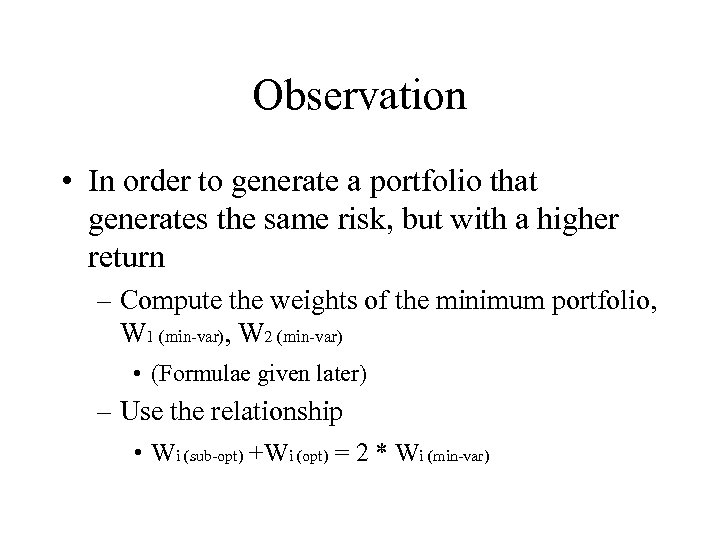Observation • In order to generate a portfolio that generates the same risk, but with a higher return – Compute the weights of the minimum portfolio, W 1 (min-var), W 2 (min-var) • (Formulae given later) – Use the relationship • Wi (sub-opt) +Wi (opt) = 2 * Wi (min-var)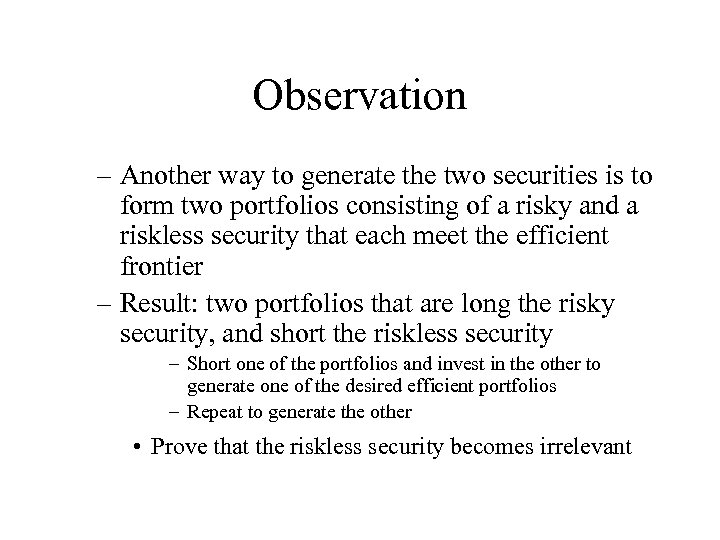Observation – Another way to generate the two securities is to form two portfolios consisting of a risky and a riskless security that each meet the efficient frontier – Result: two portfolios that are long the risky security, and short the riskless security – Short one of the portfolios and invest in the other to generate one of the desired efficient portfolios – Repeat to generate the other • Prove that the riskless security becomes irrelevant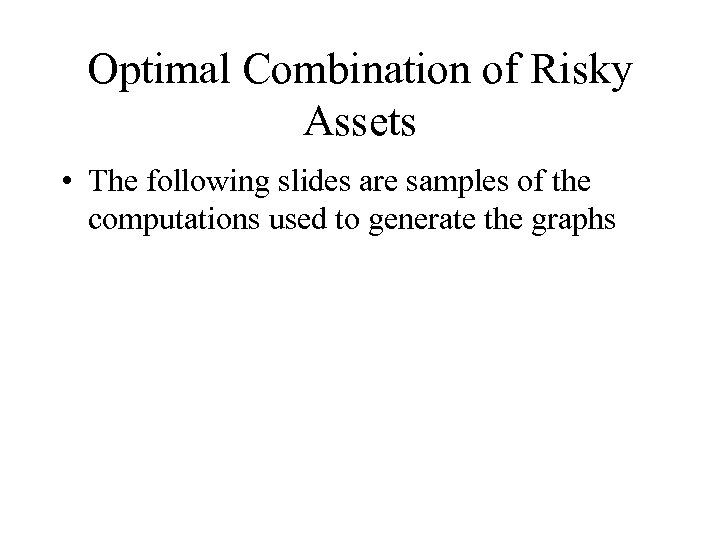Optimal Combination of Risky Assets • The following slides are samples of the computations used to generate the graphs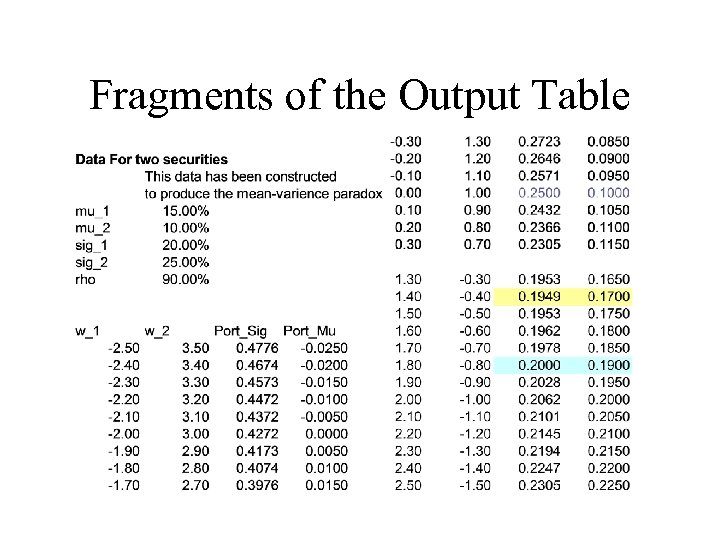Fragments of the Output Table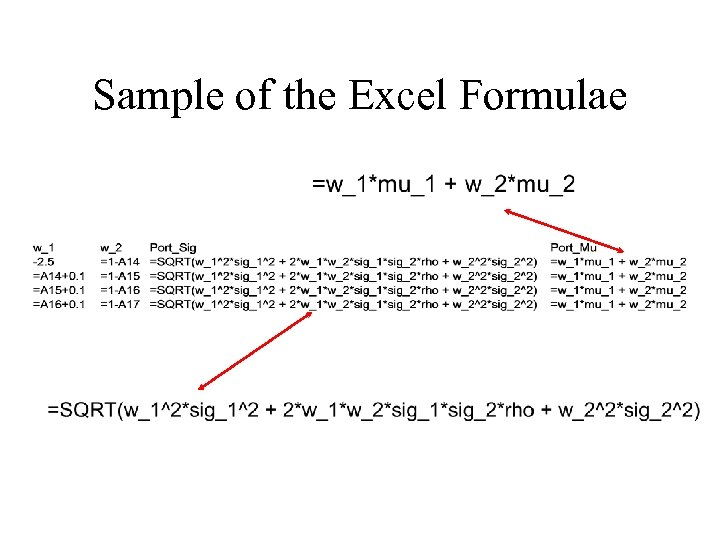Sample of the Excel Formulae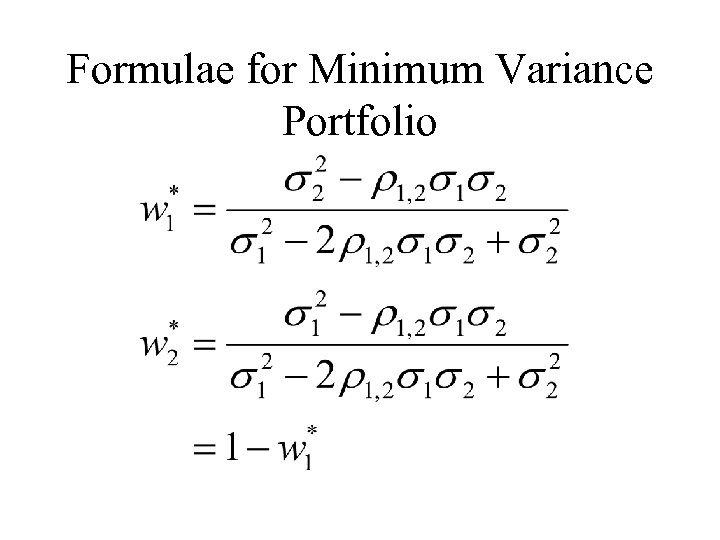Formulae for Minimum Variance Portfolio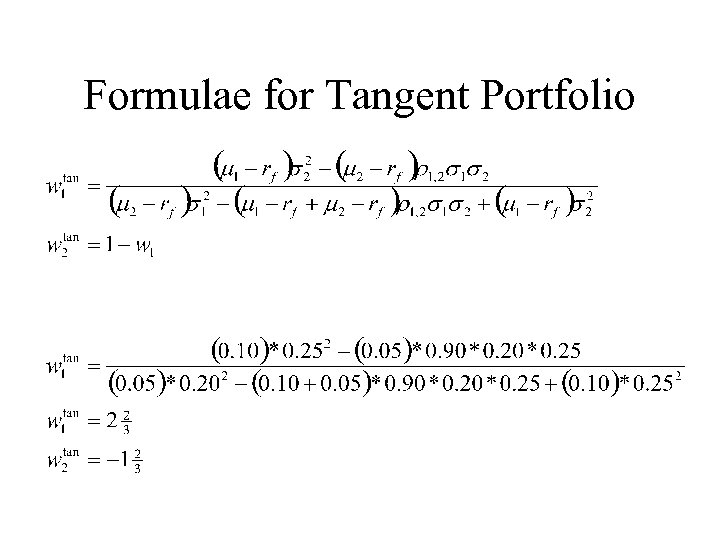Formulae for Tangent Portfolio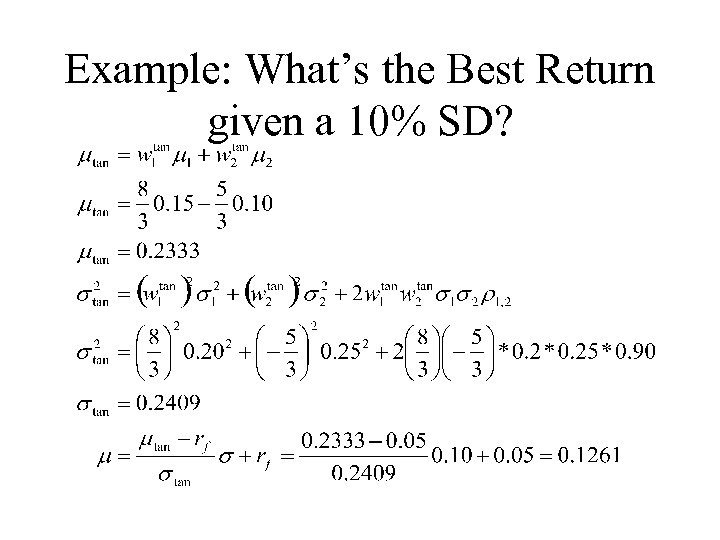Example: What’s the Best Return given a 10% SD?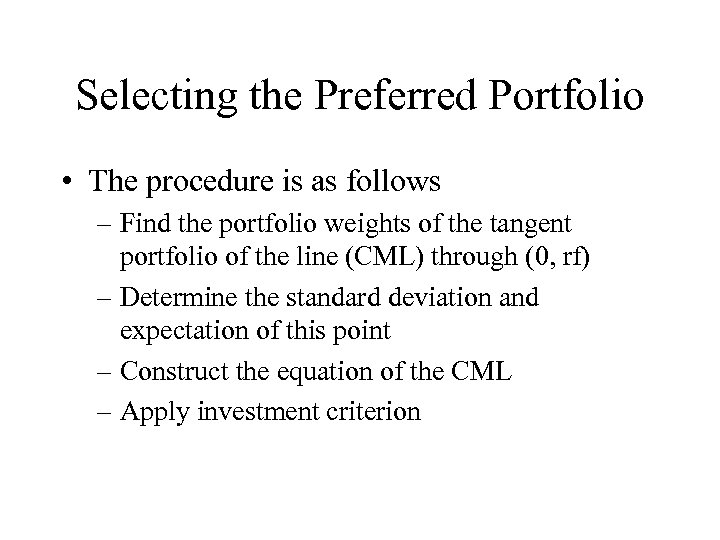Selecting the Preferred Portfolio • The procedure is as follows – Find the portfolio weights of the tangent portfolio of the line (CML) through (0, rf) – Determine the standard deviation and expectation of this point – Construct the equation of the CML – Apply investment criterion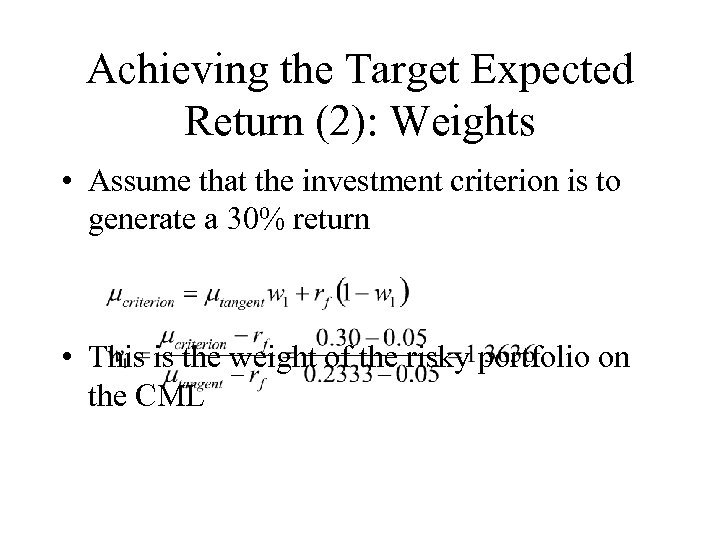Achieving the Target Expected Return (2): Weights • Assume that the investment criterion is to generate a 30% return • This is the weight of the risky portfolio on the CML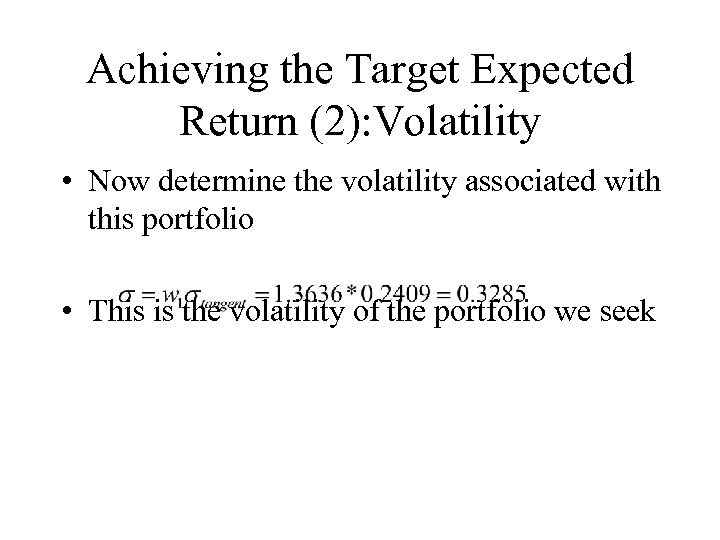Achieving the Target Expected Return (2): Volatility • Now determine the volatility associated with this portfolio • This is the volatility of the portfolio we seek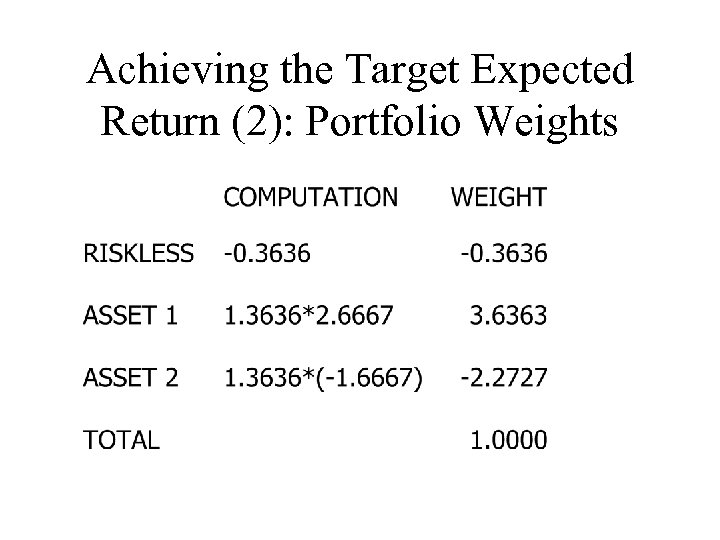Achieving the Target Expected Return (2): Portfolio Weights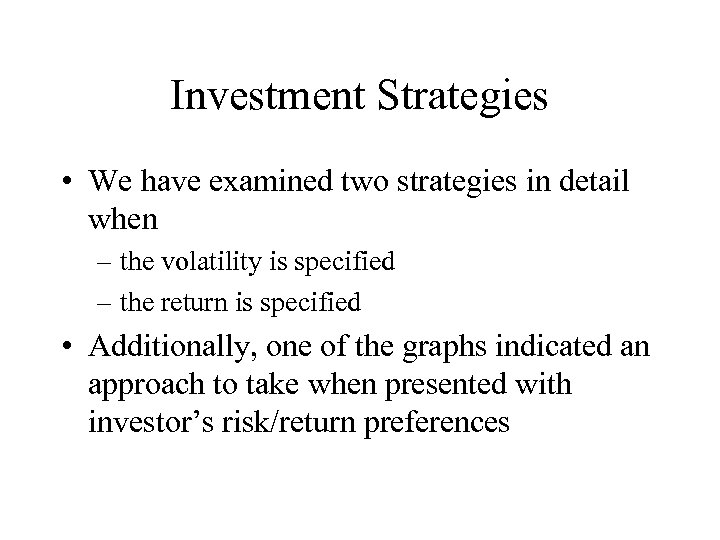Investment Strategies • We have examined two strategies in detail when – the volatility is specified – the return is specified • Additionally, one of the graphs indicated an approach to take when presented with investor’s risk/return preferences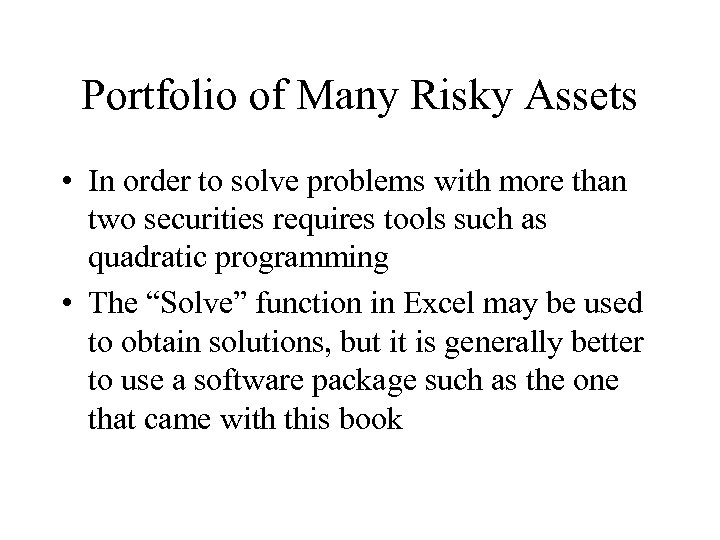Portfolio of Many Risky Assets • In order to solve problems with more than two securities requires tools such as quadratic programming • The “Solve” function in Excel may be used to obtain solutions, but it is generally better to use a software package such as the one that came with this book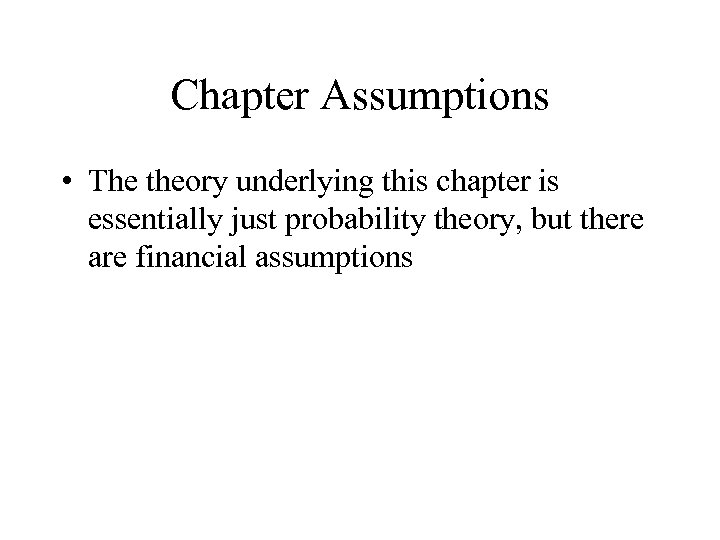Chapter Assumptions • The theory underlying this chapter is essentially just probability theory, but there are financial assumptions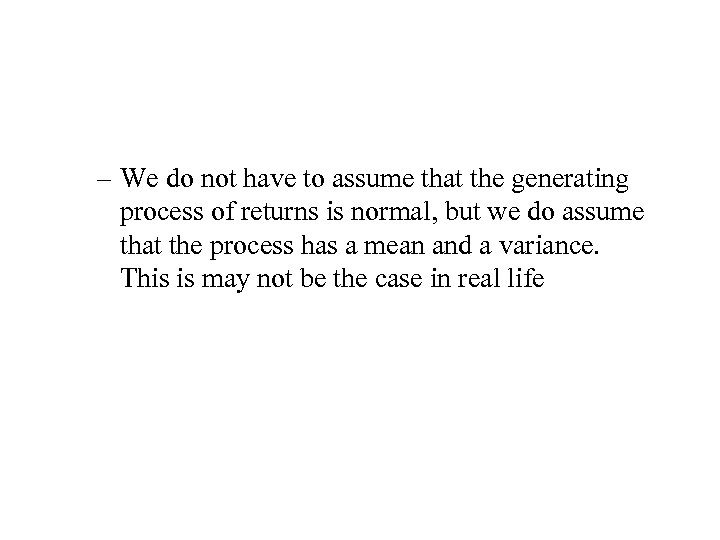– We do not have to assume that the generating process of returns is normal, but we do assume that the process has a mean and a variance. This is may not be the case in real life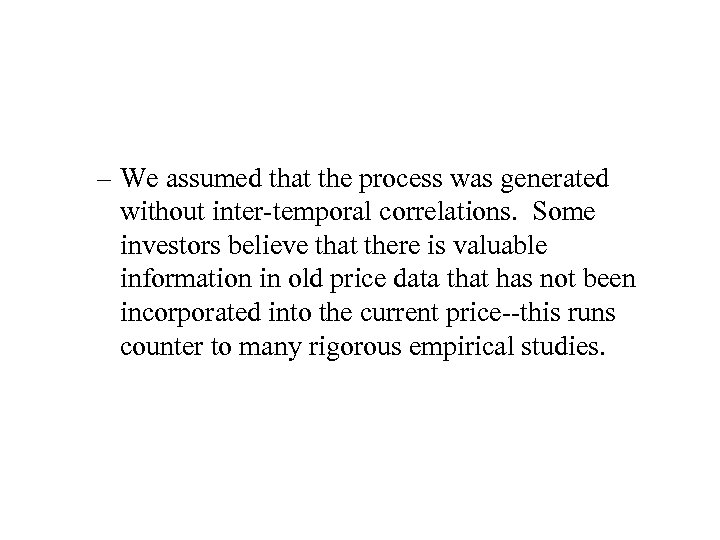– We assumed that the process was generated without inter-temporal correlations. Some investors believe that there is valuable information in old price data that has not been incorporated into the current price--this runs counter to many rigorous empirical studies.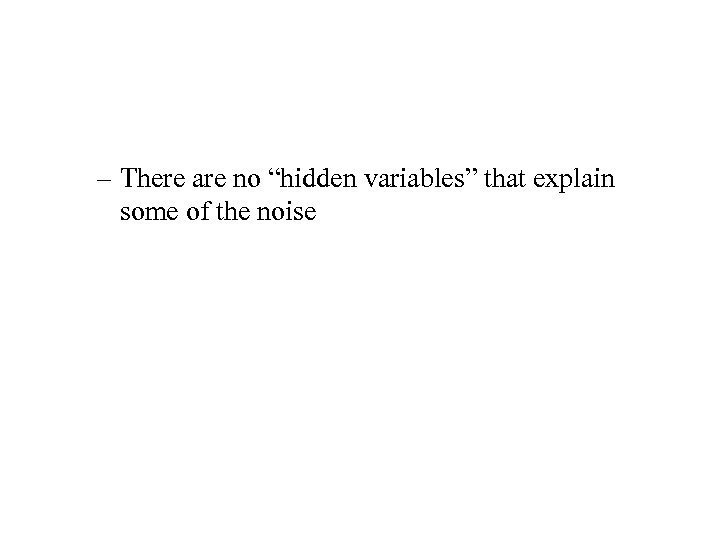– There are no “hidden variables” that explain some of the noise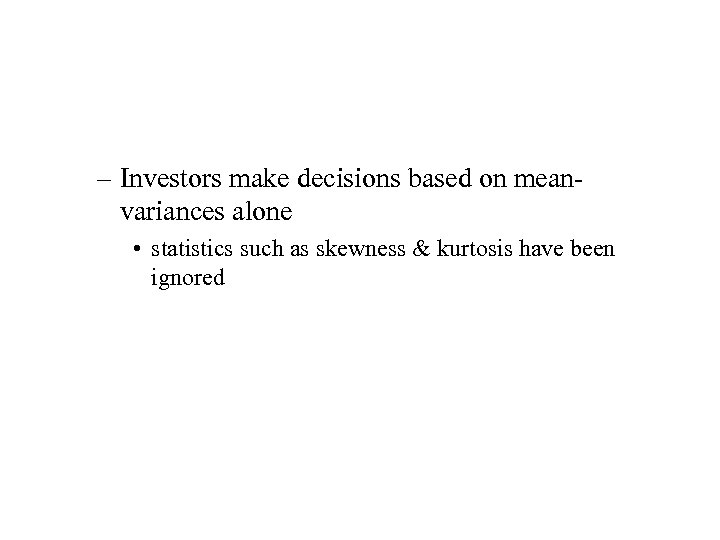– Investors make decisions based on meanvariances alone • statistics such as skewness & kurtosis have been ignored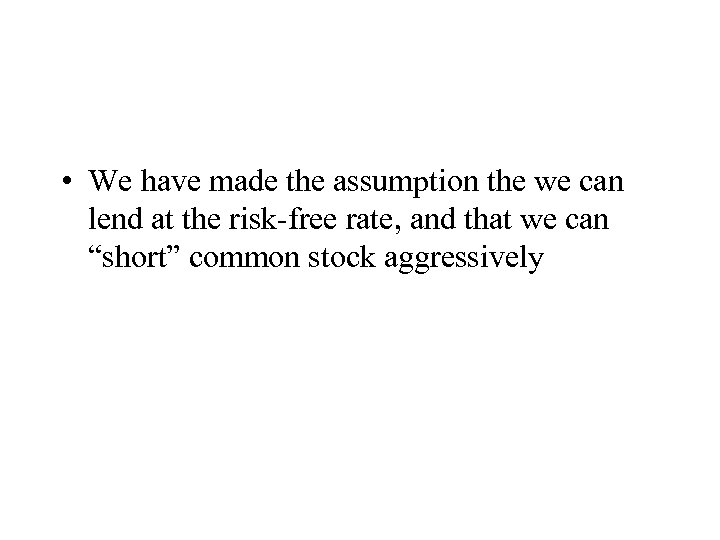• We have made the assumption the we can lend at the risk-free rate, and that we can “short” common stock aggressively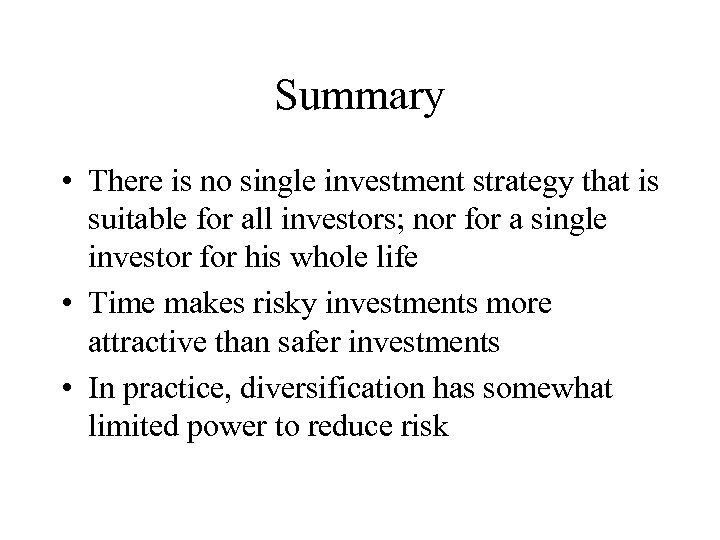Summary • There is no single investment strategy that is suitable for all investors; nor for a single investor for his whole life • Time makes risky investments more attractive than safer investments • In practice, diversification has somewhat limited power to reduce risk